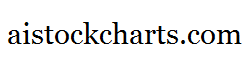Members Area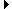Sign Up | Log In
 Free Level 2 QuotesStock AnalysisCorrelation Trading PlatformCorrelationsMembership Benefits

P1CHANGE
 Lookup a different indicator.

 Indicator Usage Description Example p1change p1change[n] OPERATOR (INDICATOR or NUMBER) , where n is a positive integer >= 0. Returns the percentage change from the opening to closing price of day n where n=0 is the last trading day, n=1 is the previous day, etc. (p1change > -1) and (p1change < 1)

Introduction:

The 'p1change' indicator is used in indicator formula construction to narrow the stock pre-screener results to include only those stocks that have a percentage change from the opening to closing price restricted to the parameters set for the p1change indicator.

Example:

The chart of Intel (INTC) below highlights days where the percentage change from the opening to closing stock price was between -1% and 1%.

 Chart of Intel (INTC) highlighting days where the percentage change from the opening to closing stock price was between -1% and 1%.

INDICATORS IN OUR DATABASE THAT INCLUDE THE P1CHANGE INDICATOR...

Click here to view all indicators that include the indicator 'p1change' (sorted by AISCORE)

 (p1change < -3) and (p2change < -3) and (p3change < -3) and (p4change < -3) and (p5change < -3)AISCORE: 6741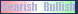Indicator ID: 060130220201(p1change < 0) and (p1change >= -1.5) and (p1change < -0.5) and (p1change >= -2) and (p1change < -1)AISCORE: 483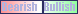Indicator ID: 060130223051(p1change > -1) and (p1change < 1)AISCORE: 1788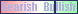Indicator ID: 060324091808(rsi > 52) and (p1range < 5) and (p1change < -2)AISCORE: 4611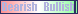Indicator ID: 060811175100(p1change > 1) and (p4range < 5) and (p1range < 8)AISCORE: 1423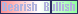Indicator ID: 060815224909 More ->